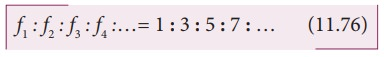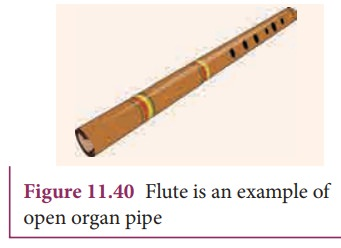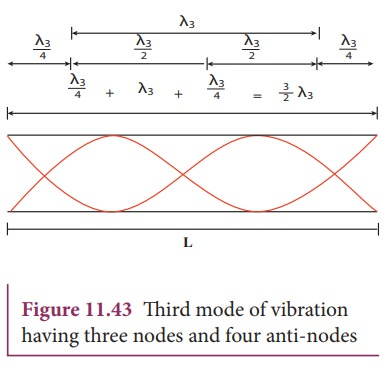Home | | Physics 11th std | Vibrations of Air Column

# Vibrations of Air Column

Musical instruments like flute, clarinet, nathaswaram, etc are known as wind instruments.

VIBRATIONS OF AIR COLUMN

Musical instruments like flute, clarinet, nathaswaram, etc are known as wind instruments. They work on the principle of vibrations of air columns. The simplest form of a wind instrument is the organ pipe. It is made up of a wooden or metal pipe which produces the musical sound. For example, flute, clarinet and nathaswaram are organ pipe instruments. Organ pipe instruments are classified into two types:

## (a) Closed organ pipes:Look at the picture of a clarinet, shown in Figure 11.36. It is a pipe with one end closed and the other end open. If one end of a pipe is closed, the wave reflected at this closed end is 180° out of phase with the incoming wave. Thus there is no displacement of the particles at the closed end. Therefore, nodes are formed at the closed end and anti-nodes are formed at open end.Let us consider the simplest mode of vibration of the air column called the fundamental mode. Anti-node is formed at the open end and node at closed end. From the Figure 11.37, let L be the length of the tube and the wavelength of the wave produced. For the fundamental mode of vibration, we have,which is called the fundamental note.

The frequencies higher than fundamental frequency can be produced by blowing air strongly at open end. Such frequencies are called overtones.

The Figure 11.38 shows the second mode of vibration having two nodes and two anti-nodes, for which we have, from example 11.20.is called first over tone, since here, the frequency is three times the fundamental frequency it is called third harmonic.

The Figure 11.39 shows third mode of vibration having three nodes and three anti-nodes.is called second over tone, and since n = 5 here, this is called fifth harmonic. Hence, the closed organ pipe has only odd harmonics and frequency of the nth harmonic is fn = (2n+1)f1. Therefore, the frequencies of harmonics are in the ratio## (b) Open organ pipes:Consider the picture of a flute, shown in Figure 11.40. It is a pipe with both the ends open. At both open ends, anti-nodes are formed. Let us consider the simplest mode of vibration of the air column called fundamental mode. Since anti-nodes are formed at the open end, a node is formed at the mid-point of the pipe.From Figure 11.41, if L be the length of the tube, the wavelength of the wave produced is given bywhich is called the fundamental note.

The frequencies higher than fundamental frequency can be produced by blowing air strongly at one of the open ends. Such frequencies are called overtones.The Figure 11.42 shows the second mode of vibration in open pipes. It has two nodes and three anti-nodes, and therefore,

L =λ2 or λ2 = L

The frequencyis called first over tone. Since n = 2 here, it is called the second harmonic.The Figure 11.43 above shows the third mode of vibration having three nodes and four anti-nodesis called second over tone. Since n = 3 here, it is called the third harmonic.

Hence, the open organ pipe has all the harmonics and frequency of nth harmonic is fn = nf1. Therefore, the frequencies of harmonics are in the ratio

f1 : f2 : f3 : f4 :…= 1 : 2 : 3 : 4 : … 11.79

### EXAMPLE 11.25

If a flute sounds a note with 450Hz , what are the frequencies of the second, third, and fourth harmonics of this pitch?. If the clarinet sounds with a same note as 450Hz, then what are the frequencies of the lowest three harmonics produced ?.

### Solution

For a flute which is an open pipe, we have

Second harmonics          f2 = 2 f1 = 900 Hz

Third harmonics   f3 = 3 f1        = 1350 Hz

Fourth harmonics f4 = 4 f1        = 1800 Hz

For a clarinet which is a closed pipe, we have

Second harmonics          f 2 = 3 f1      = 1350 Hz

Third harmonics   f 3 = 5 f1       = 2250 Hz

Fourth harmonics f 4 = 7 f1       = 3150 Hz

### EXAMPLE 11.26

If the third harmonics of a closed organ pipe is equal to the fundamental frequency of an open organ pipe, compute the length of the open organ pipe if the length of the closed organ pipe is 30 cm.

### Solution

Let l2 be the length of the open organ pipe, with l1 =30 cm the length of the closed organ pipe.

It is given that the third harmonic of closed organ pipe is equal to the fundamental frequency of open organ pipe.

The third harmonic of a closed organ pipe isThe fundamental frequency of open organ pipe isTags : Waves | Physics , 11th Physics : UNIT 11 : Waves
Study Material, Lecturing Notes, Assignment, Reference, Wiki description explanation, brief detail
11th Physics : UNIT 11 : Waves : Vibrations of Air Column | Waves | Physics